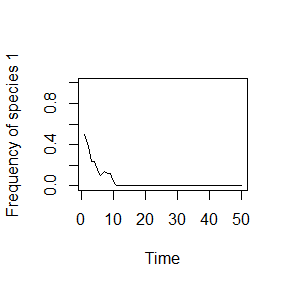## Online Box 1: R code for simulating neutral dynamics in a local, two species community.

The code here is exactly as in Box 6.1 in the book, but with annotations presented in a slightly different format.

This code runs just one simulation at a time. Online Box 2 presents code for running multiple simulations of this neutral model, as well as models with selection.

## specify initial community and time instructions
J <- 50
init.1 <- J / 2
COM <- vector(length = J)

COM[1:init.1] <- 1
COM[(init.1 + 1):J] <- 2

num.years <- 50
year <- 2

## Set up vector for data collection
freq.1.vec <- vector(length = num.years)
freq.1.vec <- init.1 / J

## run simulation
for(i in 1:(J * (num.years - 1))) {

freq.1 <- sum(COM == 1) / J
Pr.1  <- freq.1
COM[ceiling(J * runif(1))] <- sample(c(1, 2), 1, prob = c(Pr.1, 1 - Pr.1))

if (i %% J == 0){
freq.1.vec[year] <- sum(COM == 1) / J
year <- year + 1
}
}

# graph the results
plot(1:num.years, freq.1.vec, type = "l",
xlab = "Time",
ylab = "Frequency of species 1",
ylim = c(0, 1))### Description of the code. These are detailed explanations, suitable for an R beginner:

1. Define the local commmunity size, J . J is defined as an object, and <- places the number 50 in this object. Define also the initial population size of species 1, init.1. By default, the initial population size of species 2 will be J – init.1.
J <- 50
init.1 <- J / 2
1. Create an empty vector of length J to represent the community, and call it COM. Set the initial population sizes of species 1 and 2 by making elements 1 through init.1 of COM equal to 1, and the rest equal to 2.
COM <- vector(length = J)
COM[1:init.1] <- 1
COM[(init.1 + 1):J] <- 2
1. Set the number of years over which to run the simulation and define the first year to be simulated as year 2 (the initial specified community will be year 1). If we want to record output each year, as opposed to after each individual birth-death event, we need this to keep track of years in the loop.
num.years <- 50
year <- 2
1. Create an empty vector to hold the output. We only need to keep track of the frequency of species 1, so we call this vector freq.1.vec (the frequency of species 2 is 1 – the frequency of species 1). Record the initial frequency of species 1 in the first element of freq.1.vec.
freq.1.vec <- vector(length = num.years)
freq.1.vec <- init.1 / J
1. Initiate the simulation. Since each year involves J birth-death events, we need to go through the loop (i.e., repeat the birth-death cycle) J*num.years times in order to simulate the specified number of years. The variable i keeps track of how many times we’ve gone through the loop: the first time through the loop, i = 1; the second time, i = 2, and so on.
for(i in 1:(J * (num.years - 1))) {
1. Calculate the current frequency of species 1, freq.1. COM == 1 creates a vector with a “TRUE” (read quantitatively as 1) for any element equal to 1, and a “FALSE” (quantitatively zero) otherwise (in this case when it is a 2). So, taking the sum of COM == 1 gives us the current population size of species 1, and dividing by J gives the frequency. Pr.1 is the probability that an individual of species 1 is chosen to reproduce; since this model is neutral, it is equal to freq.1.
  freq.1 <- sum(COM == 1) / J
Pr.1  <- freq.1
1. Select an individual to die and replace it with an individual of the species chosen to reproduce. runif(1) draws one random number from a uniform distribution between 0 and 1, so J*runif(1) generates a random number between 0 and J. But we need an integer to select an individual from the community, and the ceiling function rounds up our random number to provide a random integer between 1 and J. This is the individual that will die. On the right hand side, we determine the species identity of the reproducing individual based on Pr.1. c(1,2) concatenates the numbers 1 and 2 together in a vector, and we sample 1 number from this vector based on the probabilities Pr.1 for species 1 and 1 – Pr.1 for species 2. Thus, we chose a 1 or a 2 to replace the dead individual.
  COM[ceiling(J * runif(1))] <- sample(c(1, 2), 1, prob = c(Pr.1, 1 - Pr.1))
1. After each sequence of J deaths, record data. i %% J returns the remainder of i divided by J, and each time that J deaths have occurred this will be equal to zero, so this is an efficient way to tell R to “stop” the program, record the frequency of species 1, and increment the year tracker by 1. The two } symbols after the snippet of code below terminate the if loop and the for loop.
  if (i %% J == 0){
freq.1.vec[year] <- sum(COM == 1) / J
year <- year + 1
}
}
1. Plot the results. 1:num.years is the data for the x-axis and freq.1.vec the data for the y-axis. type=”l” specifies a line graph, xlab and ylab the axis labels, and ylim specifies limits on the y-axis values.
plot(1:num.years, freq.1.vec, type = "l",
xlab = "Time",
ylab = "Frequency of species 1",
ylim = c(0, 1))